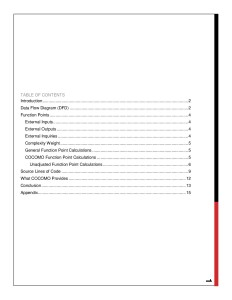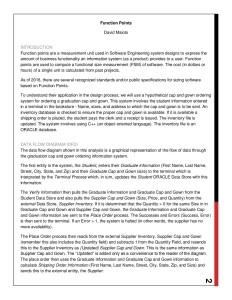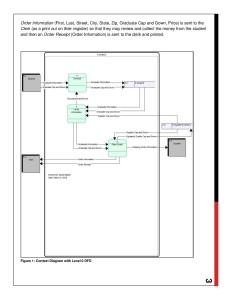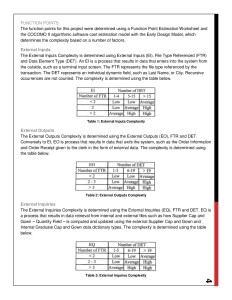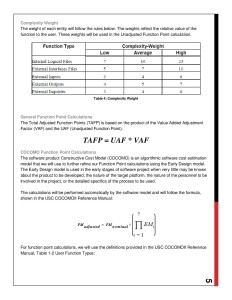# Function Points – Mathematical Analysis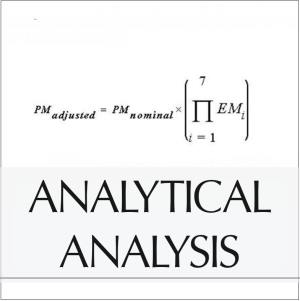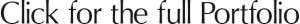This mathematical analysis by David Maiolo explains the use of function points in software engineering system designs. Function points are a unit of measurement used to express the amount of business functionality an information system provides to a user. The cost of a single unit is calculated based on past projects.

The analysis uses a hypothetical example of a cap and gown ordering system to explain how function points are used to compute a functional size measurement (FSM) of software. A data flow diagram is provided to show the flow of data through the system.

The analysis includes the use of COCOMO, an algorithmic software cost estimation model, to further refine the function point calculations. The calculations are performed using the Early Design model in COCOMO. The total adjusted function points (TAFP) are based on the product of the Value Added Adjustment Factor (VAF) and the Unadjusted Function Point (UAF).

The analysis also includes source lines of code (SLOC) for each module and total SLOC for the entire project. COCOMO can automatically estimate the maintenance activity duration and the average maintenance staffing level.

In conclusion, COCOMO provides an automated calculation of many important project development formulas. The values calculated include the total SLOC count for the project, the total estimated effort, schedule, productivity, cost, cost per instruction, full-time software programmers, and risk.

This mathematical analysis was used as a development tool in the NID (Network Image Deployment) system I created, which can also be seen on this website.

Documentation Thumbnails

Click the link at the top of the page to see the full resolution document.